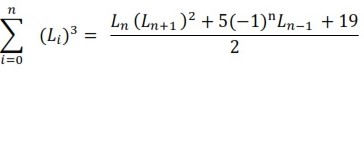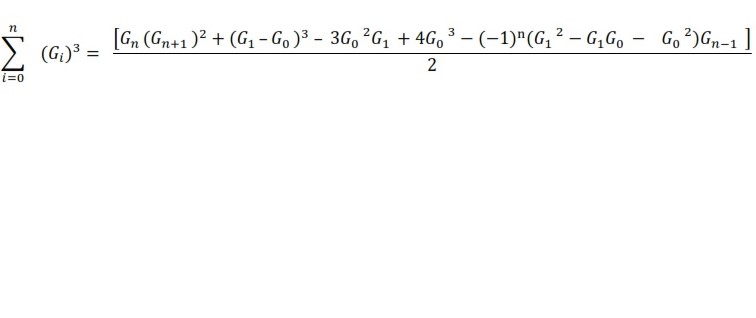## Main Article Content

Wamiliana Wamiliana Suharsono Suharsono Paustinus Edi Kristanto
DOI: https://doi.org/10.26554/sti.2019.4.2.31-35 Published Apr 27, 2019

## Abstract

Lucas and Gibonacci numbers are  two  sequences of numbers derived from a welknown numbers, Fibonacci numbers. The difference between Lucas and Fibonacci numbers only lies on the first and second elements.  The first element in Lucas numbers  is 2 and the second is 1, and nth element,  n ≥ 3 determined by similar pattern as in the Fibonacci numbers, i.e : Ln = Ln-1  + Ln-2.  Gibonacci numbers  G0 , G1 ,G2 , ...; Gn = Gn-1 + Gn-2 are generalized of Fibonacci numbers, and those numbers are nonnegative integers. If G0 = 1 and G1 = 1, then the numbers are the wellknown Fibonacci numbers, and if G0 = 2 and G1 = 1, the numbers are Lucas numbers. Thus, the difference of those three sequences of numbers only lies on the first and second of the elements in the sequences.  For Fibonacci numbers there are quite a lot identities already explored, including the sum of cubes, but there have no discussions yet about the sum of cubes for Lucas and Gibonacci numbers. In this study the sum of  cubes of Lucas and Gibonacci numbers will be discussed and showed that the sum of cubes for Lucas numbers  isand for Gibonacci numbers isAbstract 384 times PDF 247 times

## Article Details

How to Cite
WAMILIANA, Wamiliana; SUHARSONO, Suharsono; KRISTANTO, Paustinus Edi. Counting the sum of cubes for Lucas and Gibonacci numbers. Science and Technology Indonesia, [S.l.], v. 4, n. 2, p. 31-35, apr. 2019. ISSN 2580-4391. Available at: <http://sciencetechindonesia.com/index.php/jsti/article/view/162>. Date accessed: 11 july 2020. doi: https://doi.org/10.26554/sti.2019.4.2.31-35.
Section
Articles